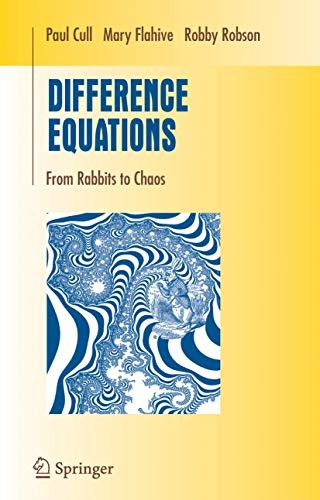• Free Shipping on all orders in Australia
• Over 7 million books in stock
• Proud to be B-Corp
• We aim to be carbon neutral by 2022
• Over 120,000 Trustpilot reviews
Item 1 of 0# Difference Equations by Paul Cull

Condition - New
\$142,39
Only 2 left

## Summary

Difference equations are models of the world around us. From clocks to computers to chromosomes, processing discrete objects in discrete steps is a common theme. This text covers the basics of difference equations and some of their applications in computing and in population biology.

## Difference Equations Summary

### Difference Equations: From Rabbits to Chaos by Paul Cull

In this new text, designed for sophomores studying mathematics and computer science, the authors cover the basics of difference equations and some of their applications in computing and in population biology. Each chapter leads to techniques that can be applied by hand to small examples or programmed for larger problems. Along the way, the reader will use linear algebra and graph theory, develop formal power series, solve combinatorial problems, visit Perron-Frobenius theory, discuss pseudorandom number generation and integer factorization, and apply the Fast Fourier Transform to multiply polynomials quickly.

The book contains many worked examples and over 250 exercises. While these exercises are accessible to students and have been class-tested, they also suggest further problems and possible research topics.

## Difference Equations Reviews

From the reviews:

The book under review is written for advanced undergraduate majors. ... This book is well written and easy to read, with a large number of exercises ... . Difference equations: from rabbits to chaos is a good text for those who want to teach an introductory course in recurrences from a collection of various classical points of view. ... anyone interested in difference equations ... will want Cull, Flahive, and Robson as a reference because of its interesting and unique collection of topics. (Shandelle M. Henson, Mathematical Reviews, Issue 2006 j)

This book is a nice addition to an already nice group of books on difference equations ... . would be especially appealing to students who like to compute. ... This book contains many interesting examples ... . There are over 250 exercises. This book could be used for an upper level undergraduate course or for a beginning graduate level course. ... it would be nice to have in one's personal library. (Allan Peterson, SIAM Review, Vol. 48 (1), 2006)

Recognizing the increasing importance of difference equation models ... the authors aimed to write a book that provides a solid foundation in the field and is accessible to undergraduates. ... this text is written in an informal style and with an 'algorithmic spirit'. ... There are many worked examples and the exercise sets are good ... . it provides an accessible introduction to the material and a firm foundation for applications in various scientific fields. (Henry Ricardo, MathDL, September, 2005)

As the authors point out in their introduction this is a textbook for undergraduate students taking introductory courses on discrete mathematics. The text is quite elementary, written for an audience with a preference for computational aspects of mathematics and modelling real world phenomena. This is also reflected by the exercises at the end of each chapter. Starting with simple examples ... the reader is lead to basic notions and theorems of basic linear algebra and analysis. Proofs are worked out accurately ... . (Stefan Hilger, Zentralblatt MATH, Vol. 1085, 2006)

This book is specifically designed for American undergraduate students studying mathematics or computer science; it is part of Springer's undergraduate texts in mathematics. ... It will be useful to have on the bookshelf, and when there is a specific difference equation in front of me it will be my first port of call. (Tony Crilly, The Mathematical Gazette, Vol. 91 (521), 2007)

Preface * Fibonacci Numbers * Homogeneous Linear Recurrence Relations * Finite Difference Equations * Generating Functions * Nonnegative Difference Equations * Leslie's Population Matrix Model * Matrix Difference Equations * Modular Recurrences * Computational Complexity * Some Nonlinear Recurrences * Appendix A: Worked Examples * Appendix B: Complex Numbers * Appendix C: Highlights of Linear Algebra * Appendix D: Roots in the Unit Circle * References * Index

NPB9780387232331
9780387232331
0387232338
Difference Equations: From Rabbits to Chaos by Paul Cull
New
Hardback
Springer-Verlag New York Inc.
2005-04-12
392
N/A
Book picture is for illustrative purposes only, actual binding, cover or edition may vary.
This is a new book - be the first to read this copy. With untouched pages and a perfect binding, your brand new copy is ready to be opened for the first time